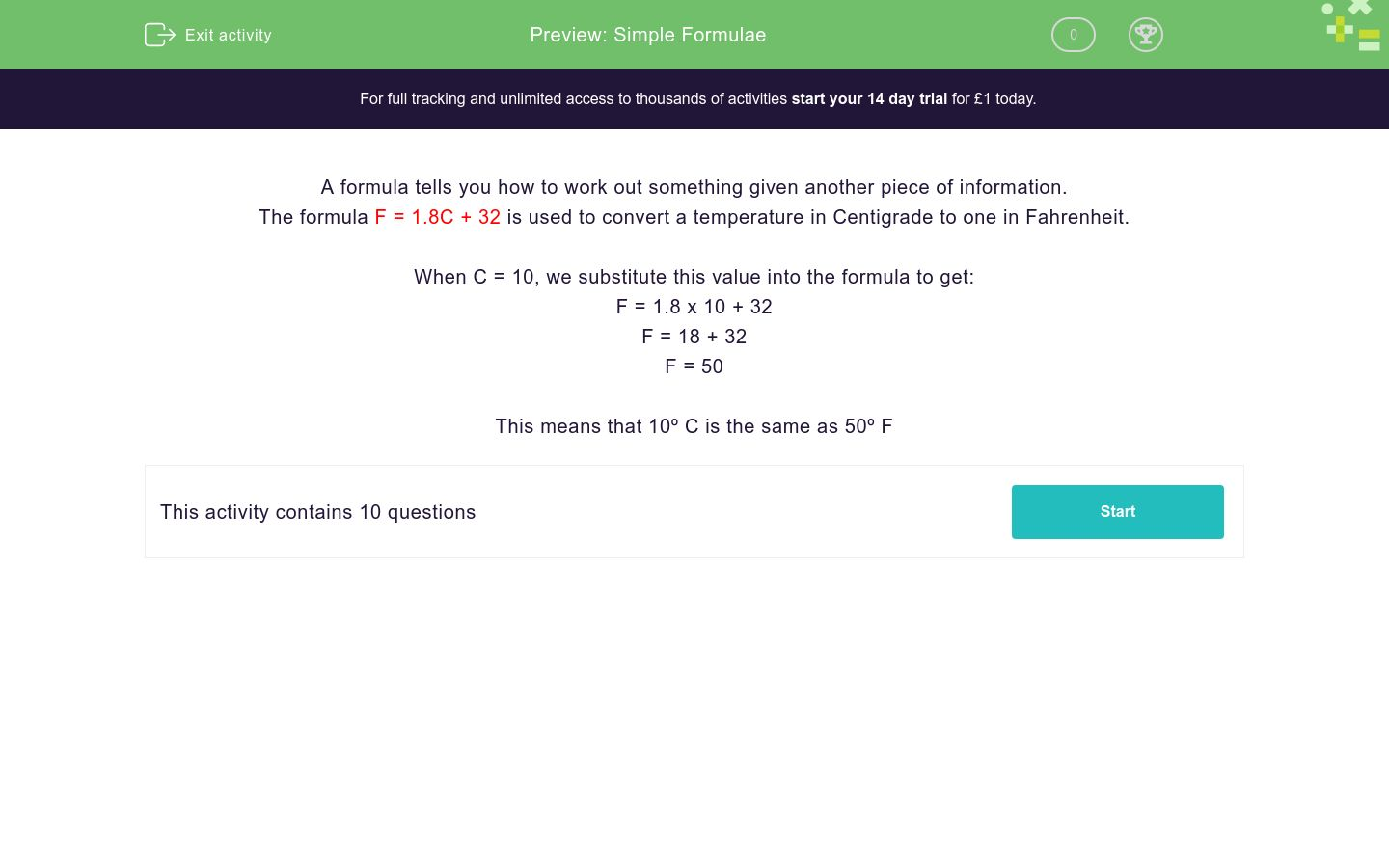# Simple Formulae

In this worksheet, students learn about and use simple algebraic formulae.Key stage:  KS 3

Curriculum topic:   Algebra

Curriculum subtopic:   Use Standard Mathematical Formulae

Difficulty level:#### Worksheet Overview

A formula tells you how to work out something given another piece of information.

The formula F = 1.8C + 32 is used to convert a temperature in Centigrade to one in Fahrenheit.

When C = 10, we substitute this value into the formula to get:

F = 1.8 x 10 + 32

F = 18 + 32

F = 50

This means that 10º C is the same as 50º F

### What is EdPlace?

We're your National Curriculum aligned online education content provider helping each child succeed in English, maths and science from year 1 to GCSE. With an EdPlace account you’ll be able to track and measure progress, helping each child achieve their best. We build confidence and attainment by personalising each child’s learning at a level that suits them.

Get started